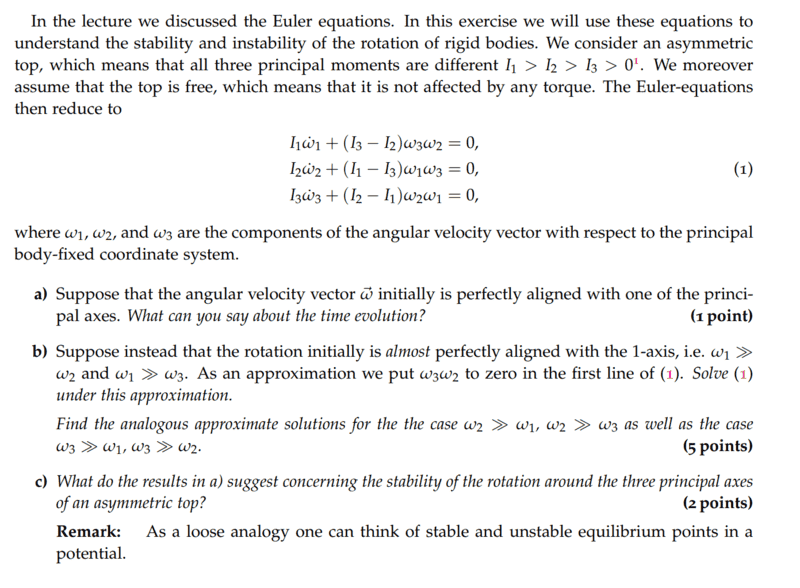# Intermediate axis theorem (Tennis racket theorem)

Lambda96
Homework Statement:
see screenshot
Relevant Equations:
Euler equation
Hi,

unfortunately, I am not getting anywhere with task bIn the lecture we had the special case that ##\vec{M}=0## , ##I_x=I_y=I , I \neq I_z## and ##\omega_z=const.##

Then the Euler equation looks like this.

$$I_x\dot{\omega_x}+\omega_y \omega_z(I_z-i_y)=0$$
$$I_y\dot{\omega_y}+\omega_z \omega_x(I_x-i_z)=0$$
$$I_z\dot{\omega_z}=0$$

With this, we then set up the following equations, where ##\Omega=\frac{I_z-I}{I}##.

$$\dot{\omega_x}+\Omega \omega_y=0$$
$$\dot{\omega_y}-\Omega \omega_x=0$$

The solution for ##\dot{\omega_x}## and ##\dot{\omega_y}## are then as follows

$$\dot{\omega_x}(t)=Acos{\Omega t + \alpha}$$
$$\dot{\omega_y}(t)=Asin{\Omega t + \alpha}$$

I then wanted to use the approximation ##\omega_1 \gg \omega_2## , ##\omega_1 \gg \omega_3## and ##\omega_3 \omega_2=0## to solve the Euler equation also according to the same recipe

The Euler equation looks like this:

$$I_1\dot{\omega_1}=0$$
$$I_2\dot{\omega_2}+\omega_1 \omega_3(I_1-I_3)=0$$
$$I_3\dot{\omega_3}+\omega_2 \omega_1(I_2-I_1)=0$$

After that I got the following equation

$$\dot{\omega}_2+\frac{(I_1-I_3)\omega_1}{I_2}\omega_3=0$$
$$\dot{\omega}_3-\frac{(I_2-I_1)\omega_1}{I_3}\omega_2=0$$

With ##\frac{(I_1-I_3)\omega_1}{I_2}=\Omega_1## and ##\frac{(I_2-I_1)\omega_1}{I_3}=\Omega_2##, the above equation is

$$\dot{\omega}_2+\Omega_1 \omega_3=0$$
$$\dot{\omega}_3- \Omega_2 \omega_2=0$$

Unfortunately, I'm not getting anywhere now, because I don't know what ##\omega_1## and ##\omega_2## have to look like to satisfy the two equations, because unfortunately I don't have ##\Omega##, but two different ones with ##\Omega_1## and ##\Omega_2##.

Homework Helper
Gold Member
After that I got the following equation$$\dot{\omega}_2+\frac{(I_1-I_3)\omega_1}{I_2}\omega_3=0$$$$\dot{\omega}_3-\frac{(I_2-I_1)\omega_1}{I_3}\omega_2=0$$
Shouldn't the second equation have a plus sign for the second term on the left side?

With ##\frac{(I_1-I_3)\omega_1}{I_2}=\Omega_1## and ##\frac{(I_2-I_1)\omega_1}{I_3}=\Omega_2##,
With these definitions, note that ##\Omega_2## is a negative number. It might be less confusing if you define ##\Omega_2 = \frac{(I_1-I_2)\omega_1}{I_3}## so that both ##\Omega_1## and ##\Omega_2## are positive.

$$\dot{\omega}_2+\Omega_1 \omega_3=0$$
$$\dot{\omega}_3- \Omega_2 \omega_2=0$$

Unfortunately, I'm not getting anywhere now, because I don't know what ##\omega_1## and ##\omega_2## have to look like to satisfy the two equations, because unfortunately I don't have ##\Omega##, but two different ones with ##\Omega_1## and ##\Omega_2##.

Take the time derivative of the first equation and then use the second equation to substitute for ##\dot \omega_3##.

•PhDeezNutz
Lambda96
Thank you TSny for your help 👍

Regarding the minus sign, I had made a mistake, I actually meant that ##\Omega_2## is negative, since ##I_1>I_2## and thus becomes negative. But I'll leave this interpretation out of the derivation for now.

The equation ##\dot{\omega_2}+\Omega_1 \omega_3=0## derived in time is as follows, actually I would have to use the product rule, but since ##\dot{\omega_1}=0## I can leave this term out directly and get

$$\ddot{\omega_2}+\Omega_1 \dot{\omega_3}=0$$

The second equation then solved for ##\dot{\omega_3}## is ##\dot{\omega_3}=-\Omega_2 \omega_2##.

Then substituting back into the first equation gives

$$\ddot{\omega_2}-\Omega_1 \Omega_2 \omega_2=0$$

Solving this differential equation then gives:

$$\omega_2=c_1e^{\sqrt{\Omega_1 \Omega_2} t}+c_2e^{-\sqrt{\Omega_1 \Omega_2} t}$$

•PhDeezNutz
Homework Helper
Gold Member
The equation ##\dot{\omega_2}+\Omega_1 \omega_3=0## derived in time is as follows, actually I would have to use the product rule, but since ##\dot{\omega_1}=0## I can leave this term out directly and get

$$\ddot{\omega_2}+\Omega_1 \dot{\omega_3}=0$$
ok

The second equation then solved for ##\dot{\omega_3}## is ##\dot{\omega_3}=-\Omega_2 \omega_2##.
ok. It appears to me that you are still defining ##\Omega_2## as ##\frac{(I_2-I_1)\omega_1}{I_3}##. So, ##\Omega_2## is a negative number. That's alright, but then you need to keep in mind that ##\sqrt{\Omega_1 \Omega_2}## is imaginary.

Then substituting back into the first equation gives

$$\ddot{\omega_2}-\Omega_1 \Omega_2 \omega_2=0$$

Solving this differential equation then gives:

$$\omega_2=c_1e^{\sqrt{\Omega_1 \Omega_2} t}+c_2e^{-\sqrt{\Omega_1 \Omega_2} t}$$
ok. The exponents on ##e## are imaginary. I think it would be nicer to rewrite this without imaginary quantities. You could use Euler's formula. Or, you could go back and define ##\Omega_2## such that it is positive and rederive the differential equation for ##\ddot \omega_2##. Then, you don't run into imaginary quantities.